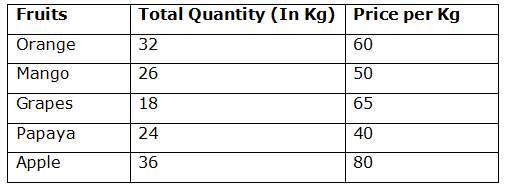# SBI PO Prelims Quantitative Aptitude Questions 2019 – Day 08

SBI PO 2019 Notification will be expected soon. It is one of the most expected recruitment among the banking aspirants. Every year the exam pattern for SBI PO has been changing. Depends upon the changing of exam pattern the questions are quite harder compare to the previous year. So the questions are in high level than the candidate’s assumption.

As per the latest trend, our IBPS Guide is providing the updated New Exam Pattern Quantitative Aptitude questions for SBI PO 2019 Day 8. Our Skilled experts were mounting the questions based on the aspirant’s needs. So candidates shall start your preparation and practice on daily basis with our SBI PO pattern quantitative aptitude questions 2019 day 8. Start your effective preparation from the right beginning to get success in upcoming SBI PO 2019.

[WpProQuiz 5069]

### Click Here for SBI PO Pre 2019 High-Quality Mocks Exactly on SBI Standard

Directions (Q. 1 – 5): Find the wrong term in the following number series?

1) 54, 28, 44, 112, 399.5, 1802.75

a) 28

b) 44

c) 112

d) 399.5

e) 1802.75

2) 506, 600, 702, 810, 930, 1056

a) 810

b) 506

c) 702

d) 930

e) 1056

3) 1656, 826, 410, 201, 94.5, 41.75

a) 826

b) 94.5

c) 410

d) 201

e) 1656

4) 36, 40, 89, 283, 1156, 5821

a) 36

b) 40

c) 89

d) 1156

e) 5821

5) 1156, 1164, 1228, 1442, 1956, 2956

a) 1164

b) 1228

c) 2956

d) 1956

e) 1442

Directions (Q. 6 – 10): Study the following information carefully and answer the given questions:

The following table shows the different number of fruits sold by a shop in a certain day and the price per kg also given.Note: Total cost = Total quantity × Price per kg

6) Find the difference between the total cost received by selling orange to that of Apple in a day?

a) Rs. 960

b) Rs. 840

c) Rs. 1020

d) Rs. 1150

e) None of these

7) Find the total cost received by selling papaya in a whole week (i.e., 7 days)? (The selling price per kg for a whole week is same as the price given in the table)

a) Rs. 6280

b) Rs. 7150

c) Rs. 5940

d) Rs. 6720

e) None of these

8) Find the average amount received by selling Grapes, Papaya and Apple in a day?

a) Rs. 2350

b) Rs. 1670

c) Rs. 1940

d) Rs. 2180

e) None of these

9) If the same quantity of Oranges sold by the shop in the whole month at the same rate (i.e., 31 days), then find the total amount received by selling the orange in the month?

a) Rs. 63760

b) Rs. 47440

c) Rs. 59520

d) Rs. 51280

e) None of these

10) Total cost received by selling Grapes in a day is what percentage of total cost received by selling Apple in a day?

a) 52.25 %

b) 66.75 %

c) 48.125 %

d) 40.625 %

e) 33.5 %

Direction (1-5) :

The correct series is,

54, 28, 44, 113, 399.5, 1802.75

The pattern is, *0.5 + 1, *1.5 + 2, *2.5 + 3, *3.5 + 4, *4.5 + 5,..

The wrong term is, 112

The correct series is,

506, 600, 702, 812, 930, 1056

The pattern is, 232 – 23, 252 – 25, 272 – 27, 292 – 29, 312 – 31, 332 – 33,…

The wrong term is, 810

The correct series is,

1656, 826, 410, 201, 95.5, 41.75

The pattern is, *1/2 – 2, *1/2 – 3, *1/2 – 4, *1/2 – 5, *1/2 – 6,…

The wrong term is, 94.5

The correct series is,

36, 40, 89, 283, 1157, 5821

The pattern is, *1 + 22, *2 + 32, *3 + 42, *4 + 52, *5 + 62,…

The wrong term is, 1156

The correct series is,

1156, 1164, 1228, 1444, 1956, 2956

The pattern is, +23, +43, +63, +83, +103,…

The wrong term is, 1442

Direction (6-10) :

The total cost received by selling orange in a day

= > 32*60 = Rs. 1920

The total cost received by selling Apple in a day

= > 36*80 = Rs. 2880

Required difference = 2880 – 1920 = Rs. 960

The total cost received by selling papaya in a whole week

= > 24*40*7 = Rs. 6720

Total amount received by selling Grapes, Papaya and Apple in a day

= > (18*65) + (24*40) + (36*80)

= > 1170 + 960 + 2880 = Rs. 5010

Required average = 5010/3 = Rs. 1670

Total amount received by selling the orange in the whole month

= > 32*60*31 = Rs. 59520

Total cost received by selling Grapes in a day

= > (18*65) = Rs. 1170

Total cost received by selling Apple in a day

= > (36*80) = Rs. 2880

Required % = (1170/2880)*100 = 40.625 %